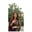Related Tags

communitycreator

# What are Kohonen Self-Organizing Maps?Arooj Fatima

The Kohonen Self-Organizing Mapis named after the researcher Dr. Teuvo Kohonen.

A Kohonen Self-Organizing Map is a map that is used for maintaining neighbor topology. It is referred to as a neural network that is trained by competitive learning.

## Neighbor topologies

Bellow are the two most used topologies in the Kohonen Self-Organizing Map.

### Rectangular grid

The following explains the rectangular grid topology:

• In the distance of 2 grids: 24 nodes
• In the distance of 1 grid: 16 nodes
• In the distance 0 grid: 8 nodes

There is a total difference of $8$ nodes between each grid.

## Hexagonal topology

The following explains the hexagonal grid topology:

• In the distance of 2 grids: 18 nodes
• In the distance of 1 grid: 12 nodes
• In the distance 0 grid: 6nodes

There is a total difference of 8 nodes between each grid.

Each node has its own coordinates after clustering, which can be calculated with the Pythagorean theorem using the Euclidean distance between the two nodes.

## Structure

A Kohonen Self-Organizing Map is different from common artificial neural networks in its:

• architecture properties
• algorithmic properties

A Kohonen Self-Organizing Map consists of a single layer linear 2D grid of neurons. The nodes do not know the values of their neighbors. The weights of links are updated as a function of the given inputs. However, all the nodes on the grid are directly linked to the input vectors.

### Algorithm

#### Variables used in the algorithm

• x = input vector
• x(t) =input vector’s occurrence at iteration t
• n = total number of iterations a network can perform
• t = current iteration
• i = row coordinate
• j = column coordinate
• λ = time constant
• d = distance between a node and the BMU
• w = weight vector
• w_ij = weight of the links between the nodes i,j
• β_ij = neighborhood function, representing and decreasing a node i, j distance from the BMU
• σ(t) = radius of the neighborhood function, it decides how far neighbor nodes are inspected in the 2D grid when updating vectors. It decreases over time.

#### Step 1

• Every node weight is initialized to a random value.

#### Step 2

• Select a random input vector.

#### Step 3

• Determine the Euclidean distance between the weight vector and the input vector connected with the first node considering t, i, j =0.

#### Step 4

• Note the node that generates the smallest distance.

#### Step 5

• Repeat steps 3 and 4 for all nodes.

#### Step 6

• Determine the complete BMU, i.e., node with the smallest distance from each already determined node.

#### Step 7

• Calculate βij(t) its radius σ(t) of BMU in Kohonen Self-Organizing Map.

#### Step 8

• Repeat for all nodes in the BMU neighborhood.

#### Step 9

• Update the weight vector of the first node in the neighborhood of the BMU by including a fraction of the difference between the input vector and the weight of the neuron.

#### Step 10

• Repeat the iterations until the total number of iterations are completed.

RELATED TAGS

communitycreator

CONTRIBUTORArooj Fatima
RELATED COURSES

View all Courses

Keep Exploring

Learn in-demand tech skills in half the time